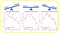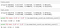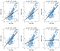# Sources of Error in Machine Learning

## The solution to a machine learning problem is not unique. The predictive power of a model depends on the experience of the data scientist in dealing with sources of error

There is no such thing as a perfect machine learning model. A model’s overall reported error has incorporated into it contributions from the following sources:

Data collection can produce errors at different levels. For instance a survey could be designed for collecting data. However, individuals participating in the survey may not always provide the right information. For instance a participant may enter wrong information about their age, height, marital status, income, etc. Error in data collection could also occur when there is error in the system designed for recording and collecting the data, for instance a faulty sensor in a thermometer could cause the thermometer to record erroneous temperature data.

Storing data could lead to error as some data could be save incorrectly or part of the data could be lost during the storage process.

Retrieving data can also produce error, as some part of the data may be missing or could be corrupted.

Often, the removal of samples or dropping of entire feature columns is simply not feasible, because we might lose too much valuable data. In this case, we can use different interpolation techniques to estimate the missing values from the other training samples in our dataset. One of the most common interpolation techniques is mean imputation, where we simply replace the missing value by the mean value of the entire feature column. Other options for the imputing missing values are median or most frequent (mode), where the latter replaces the missing values by the most frequent values. This is useful for imputing categorical feature values. Another imputation technique that can also be used is median imputation. Whatever imputation method you employ in your model, you have to keep in mind that imputation is only an approximation, and hence can produce error in the final model.

In order to bring features to the same scale, we could decide to use either normalization or standardization of features. Most often we assume data is normally distributed and default towards standardization, but that is not always the case. It’s important that before deciding weather to use either standardization or normalization, you first take a look at how your features are distributed. If the feature tends to be uniformly distributed, then we may use normalization (MinMaxScaler). If the feature is approximately Gaussian, then we can use standardization (StandardScaler). Again, note that whether you employ normalization or standardization, these are also approximative methods and are bound to contribute to the overall error of the model.

This occurs when too few features are used in training the model. In this case the model is overly simply or underfitted. The advantage of building a model using a lower dimensional dataset lies in the fact that the final model will be simple and easy to interpret. Also a model built on a lower dimensional space containing fewer features is easy to execute (requires less computational time for training, testing, and evaluation).

This occurs when too many features are used in training the model so that the model captures both real and random effects. Generally, a model trained on a very high dimensional dataset is too complex and difficult to interpret. It is always good to find the right balance between Bias Error (underfitted) and Variance Error (overfitted) as illustrated below:Illustration of bias error (underfit) and variance error (overfit). Image source: Simplicity vs Complexity in Machine Learning — Finding the Right Balance, Benjamin O. Tayo

This error arises from the inherent random nature of the dataset. Random error can be evaluated using k-fold cross-validation. In k-fold cross-validation, the dataset is randomly partitioned into training and testing sets. The model is trained on the training set and evaluated on the testing set. The process is repeated k-times. The average training and testing scores are then calculated by averaging over the k-folds. Here is the k-fold cross-validation pseudocode:

Here is a sample output from a 10-fold cross-validation calculation:Sample R2 outputs from k-fold cross-validation calculation. Source: Hands-on k-fold Cross-validation for Machine Learning Model Evaluation — Cruise Ship Dataset, Benjamin O. Tayo

We see from the output above that the R2 values for the train and test scores are pretty consistent. This means that random variability in the dataset is minimal.

This error arises from using the wrong hyperparameter values in your model. It is important that you train your model against all hyperparameters in order to determine the model with optimal performance. A good example of how the predictive power of a model depends on hyperparameters can be found in the figure below (source: Bad and Good Regression Analysis).Regression analysis using different values of the learning rate parameter. Source: Bad and Good Regression Analysis, Published in Towards AI, February 2019, by Benjamin O. Tayo.

From the figure above, we see that the reliability of our model depends on hyperparameter tuning. If we just pick a random value for the learning rate such as eta = 0.1, this would lead to a poor model. Choosing a value for eta too small, such as eta = 0.00001 also produces a bad model. Our analysis shows that the best choice is when eta = 0.0001, as can be seen from the R-square values.

More examples of hyperparameters used in the scikit-learn package are given below:

`Perceptron(n_iter=40, eta0=0.1, random_state=0)train_test_split( X, y, test_size=0.4, random_state=0)LogisticRegression(C=1000.0, random_state=0)KNeighborsClassifier(n_neighbors=5, p=2, metric='minkowski')SVC(kernel='linear', C=1.0, random_state=0)DecisionTreeClassifier(criterion='entropy',                        max_depth=3, random_state=0)Lasso(alpha = 0.1)PCA(n_components = 4)`

This error arises from the type of machine learning algorithm selected. For example, suppose we would like to build a machine learning model for binary classification. There are lots of classification algorithms to select from such as:

`LogisticRegression()KNeighborsClassifier()SVC()DecisionTreeClassifier()RandomForestClassifier()GaussianNB()`

One way to assess model selection error would be to implement each of the algorithms above and select the one with the best performance (e.g. best R2 score or AUC value). Another method would be to perform an ensemble average where the overall R2 score can be calculated by averaging over the R2 scores from all the classifiers used.

In summary, we’ve discussed 10 possible sources of error in machine learning. Generally, the predictive power of a model depends on the experience of the individual building the model. When building a model, it is important we keep in mind the possible sources of error. The best way to reduce error in a model is to tune the model against all model parameters and hyperparameters. Then select the parameters with the optimal performance. No two machine learning projects are the same. So make sure you study your dataset carefully, and identify different effects that can produce error in your model.

Written by

## Towards AI

#### Towards AI, is the world’s fastest-growing AI community for learning, programming, building and implementing AI.

Welcome to a place where words matter. On Medium, smart voices and original ideas take center stage - with no ads in sight. Watch
Follow all the topics you care about, and we’ll deliver the best stories for you to your homepage and inbox. Explore
Get unlimited access to the best stories on Medium — and support writers while you’re at it. Just \$5/month. Upgrade